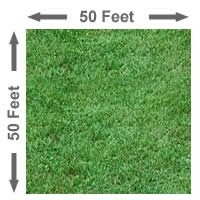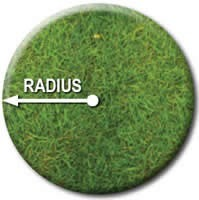# How To Calculate Square Feet Of A Planting Area

·  Page 1
Here you will find a calculator useful for determining square feet in a given area.
by Brett · All Zones · Calculations · 0 Comments · March 09, 2011 · 23,895 views

## Calculating Square Feet in Square and Circle Areas

Before using the calculation charts to determine how much mulch, sod grass, or how many plants you might need to fill a given area you will need to measure and determine the total square footage of the area to be covered or planted.

### Determining Total Square Footage of a Square or Rectangular AreaSimply multiply the length of the area by the width of the area. For example, if the length of a square area is 50 feet and the width of the area is 50 feet the equation would look like this:

50' x 50' = 2,500 Total Square Feet

Here is the equation for a rectangular area measuring 100 feet long by 25 feet wide:

100' x 25' = 2,500 Total Square Feet

### To determine the square footage of a circular area.

The formula for determining the total square feet in a circular area is: A = Pi x R2 (Area = Pi x R-squared). This formula may mean nothing to you so here's a explanation:To determine the total square footage in a circular area you will first need to determine the radius of the circle. The radius of the circle is the distance from the center of the circle to the outside (perimeter) of the circle, as shown to right. Once you know the length of the radius of a circle multiply the radius by 2. Then multiply this figure by 3.1416 to determine total square feet in the circular area.

Here is the equation for a circle that has a radius of 20 feet.

3.1416 x 20' x 20' = 1256 Total Square Feet

Here is the equation for a circle that has a radius of 5 feet:

3.1416 x 5' x 5' = 78.54 Total Square Feet

#### Meet The Author

Gardenality Genius · More Articles By Brett »